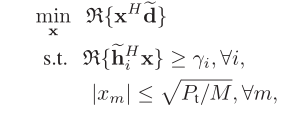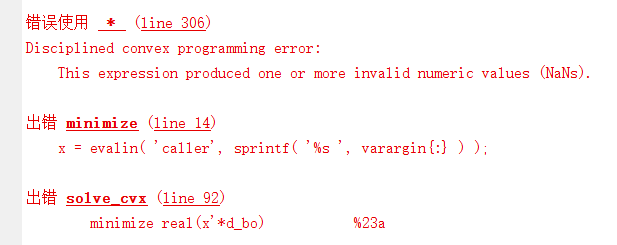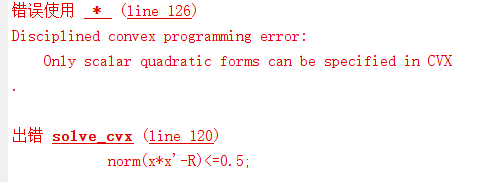# How can I solve this problem?

I want to solve a problem like thisHere is my cvx code
cvx_begin
variable x(para.M,1) complex
minimize real(x’d_bo) %23a
subject to
for k=1:2
para.K
hhh=h_i(:,k);
real((hhh)’*x)>=beita; %23b
end
for kk1=1:para.M
abs(x(kk1))<=sqrt(para.Pt/para.M); %23c
end
cvx_end

Here is error information.The error message gives you a good clue. `d_bo` apparently has at least one `NaN`. CVX does not allow `NaN` in input data (for good reason!!!).

`any(isnan(d_bo))` presumably outputs logical 1, meaning there is a tleast one `NaN` in `d_bo`.

Where did you get `d_bo` from? is that the output of a failed optimization?

Thank you for your help.And then I have another question.
Here is my cvx code
cvx_begin
variable x(para.M,1) complex
minimize real(x’d_bo) %23a
subject to
for k=1:2
para.K
hhh=h_i(:,k);
real((hhh)‘x)>=beita; %23b
end
for kk1=1:para.M
abs(x(kk1))<=sqrt(para.Pt/para.M); %23c
end
norm(x
x’-R)<=0.5;
cvx_end

Here is error information.You can only compute the norm of an affine expression. x*x’-R is clearly not an affine expression.

Expanding on @Erling’s comment, that is the matrix (operator) two norm (of a matrix argument). I.e., `maximum singular value of (x*x'-R ) <= 0.5`. That would be a convex constraint, and allowed in CVX, if the matrix argument were affine, which as @Erling pointed out, it is not. If that is the constraint you really want, it is non-convex. But is that really the constraint you want?